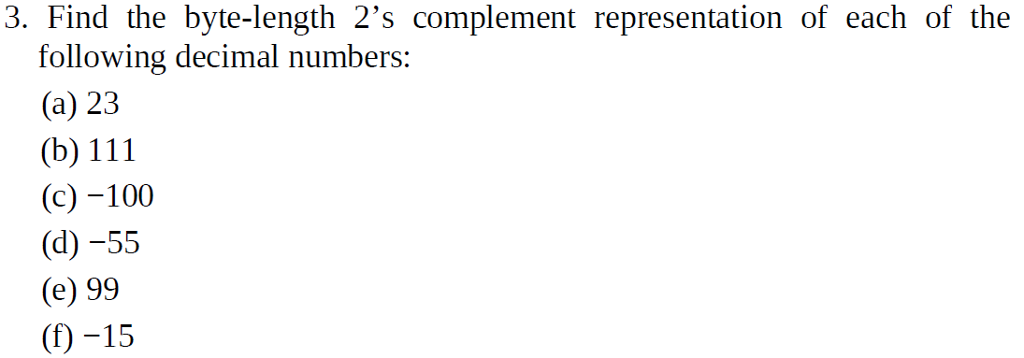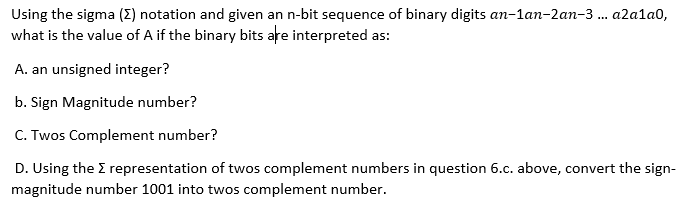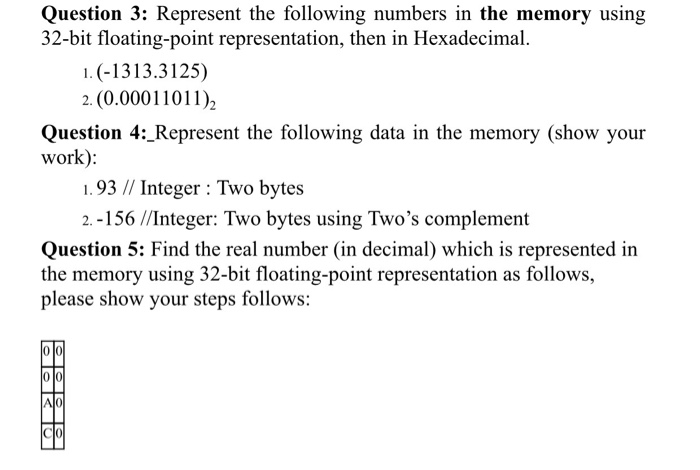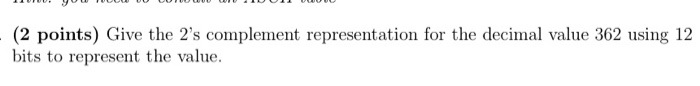Question

# 1. What is the representation of -9 in ten’s complement using two decimal digits?

1. What is the representation of -9 in ten’s complement using two decimal digits?

2. What is the representation of -9 in ten’s complement using three decimal digits?

3. Which of the following is represented as 11111100 in two’s complement ?

A. -2

B. -4

C. -6

D. 252

E. -252

1) 10s complement means 9s complement of a decimal no. + adding 1 to least significant bit

so for -9 ............. the 10s complement up to 2 decimals ...........9.99-(-9)=18.99 is 9s complement .

2) 10s   complement up to 3 decimals ...........for -9 is 9.999-(-9)=18.999 is 9s complement.

3) 11111100 is in 2s complement

so first do 1s complement to this(11111100).........is 00000011 and now add 1 to gets its original number ..

0 0 0 0 0 0 1 1

+ 1

0 0 0 0 0 1 0 0

so we got 00000100 as the answer so converting it to decimal as 0*20+0*21+1*22+0+0+0+0+0=4 as its a negative number we indicate it with negative sign

#### Earn Coins

Coins can be redeemed for fabulous gifts.

Similar Homework Help Questions
• ### 8. Using 4 bits and two’s complement representation , what is the binary representation of the...

8. Using 4 bits and two’s complement representation , what is the binary representation of the following signed decimal values; a) +6 b) -3

• ### (1) Convert this Hexadecimal to Binary, Octal and Decimal : ABCDEF (2) how the representation of...

(1) Convert this Hexadecimal to Binary, Octal and Decimal : ABCDEF (2) how the representation of each of these numbers in both two’s complement and sign magnitude formats. Use the following assumptions: ● Assume that the sign magnitude number should be represented in the fewest number of bits possible. ● Assume that the sign bit for negative sign magnitude numbers should be a 1. ● Assume that the two’s complement numbers should be 8 bit numbers. 1. 108 2. -65

• ### 4. What decimal value does the 8-bit binary number 10011110 have if:

4. What decimal value does the 8-bit binary number 10011110 have if: a) It is interpreted as an unsigned number? b) It is on a computer using signed-magnitude representation? c) It is on a computer using ones complement representation? d) It is on a computer using twos complement representation? 5. Given the following two binary numbers: 11111100 and 01110000. a) Which of these two numbers is the larger unsigned binary number? b) Which of these two is the larger when it is being...

• ### Using a two's complement representation of 10 bits, what is the maximum decimal value that can...

Using a two's complement representation of 10 bits, what is the maximum decimal value that can be stored?______________

• ### 3. Find the byte-length 2's complement representation of each of the following decimal numbers (a) 23...3. Find the byte-length 2's complement representation of each of the following decimal numbers (a) 23 (b) 111 (c) -100 (d) -55 (e) 99 (f) -15

• ### 2. Convert the following two's complement binary numbers to decimal numbers. These numbers are integers.

2. Convert the following two's complement binary numbers to decimal numbers. These numbers are integers. a. 110101 b. 01101010 c. 10110101 3. Convert the following decimal numbers to 6-bit two's complement binary numbers and add them. Write the results in 6-bit two's complement binary number format and decimal number format. Indicate whether or not the sum overflows a 6-bit result. a. 14 + 12 b. 30 + 2 C. -7 + 20 d. -18-17 4. What is the signed/magnitude and two's complement range of...

• ### Using the sigma (E) notation and given an n-bit sequence of binary digits an-lan-2an-3 ... a2alao,...Using the sigma (E) notation and given an n-bit sequence of binary digits an-lan-2an-3 ... a2alao, what is the value of A if the binary bits are interpreted as: A. an unsigned integer? b. Sign Magnitude number? C. Twos Complement number? D. Using the representation of twos complement numbers in question 6.c. above, convert the sign- magnitude number 1001 into twos complement number.

• ### Question 3: Represent the following numbers in the memory using 32-bit floating-point representation, then in Hexadecimal....Question 3: Represent the following numbers in the memory using 32-bit floating-point representation, then in Hexadecimal. 1. (-1313.3125) 2.(0.00011011) Question 4:_Represent the following data in the memory (show your work): 1.93 // Integer : Two bytes 2.-156 //Integer: Two bytes using Two's complement Question 5: Find the real number (in decimal) which is represented in the memory using 32-bit floating-point representation as follows, please show your steps follows: JOJO AOL

• ### 1 . TEODO010000 - (2 points) Give the 2's complement representation for the decimal value 362...1 . TEODO010000 - (2 points) Give the 2's complement representation for the decimal value 362 using 12 bits to represent the value.

• ### What is the decimal value of this 32-bit two’s complement number? 1111 1111 1111 1111 1111...

What is the decimal value of this 32-bit two’s complement number? 1111 1111 1111 1111 1111 1111 1111 1000 a. -4 b. -8 c. -16 d. 18,446,744,073,709,551,609 A page table is indexed with the __________ from the virtual address. a. page offset b. page number c. page table register d. none of the above.